Author: Oscar Cronquist Article last updated on April 28, 2021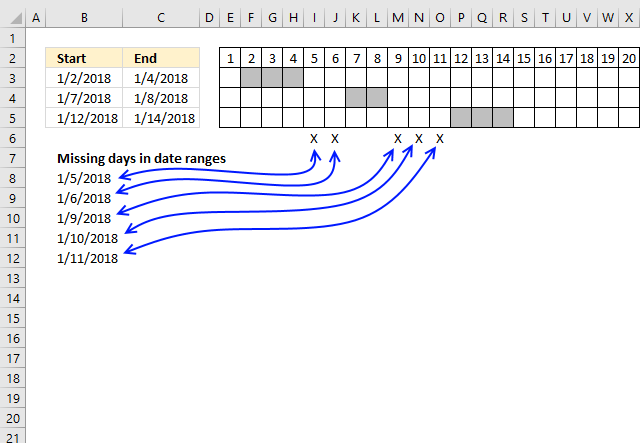The formula in cell B8, shown above, extracts dates not included in the specified date ranges, in other words, dates that are between date ranges.

I have also built a small calendar using conditional formatting to show exactly where the missing dates are. The formula below works fine with overlapping date ranges.

I will explain the formula in this article and there will also be a file for you to download.

#### What's on this webpage

Array formula in cell B8:

=SMALL(IF(COUNTIFS(\$B\$3:\$B\$5, "<="&ROW(INDIRECT(MIN(\$B\$3:\$C\$5)&":"&MAX(\$B\$3:\$C\$5))), \$C\$3:\$C\$5, ">="&ROW(INDIRECT(MIN(\$B\$3:\$C\$5)&":"&MAX(\$B\$3:\$C\$5))))=0, ROW(INDIRECT(MIN(\$B\$3:\$C\$5)&":"&MAX(\$B\$3:\$C\$5)))), ROWS(\$A\$1:A1))

To enter an array formula, type the formula in a cell then press and hold CTRL + SHIFT simultaneously, now press Enter once. Release all keys.

The formula bar now shows the formula with a beginning and ending curly bracket telling you that you entered the formula successfully. Don't enter the curly brackets yourself.

Now copy cell B8 and paste as far as needed to cells below.

Update 27th April 2021 - Excel 365 formula:

=LET(y, \$B\$3:\$C\$5, x, SEQUENCE(MAX(y)-MIN(y), , MIN(y)), SMALL(IF(COUNTIFS(\$B\$3:\$B\$5, "<="&x, \$C\$3:\$C\$5, ">="&x)=0, x), ROWS(\$A\$1:A1)))

Back to top

## 1. How to adjust cell references in the array formula to your worksheet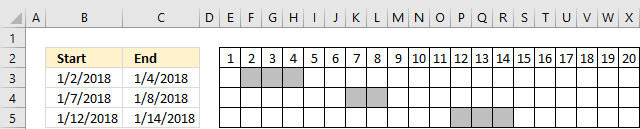Cell range \$B\$3:\$B\$5 is the start dates of the date ranges and  \$C\$3:\$C\$5 contains the end dates.

\$B\$3:\$C\$5 contains both start and end dates of your date ranges. Adjust these accordingly to your worksheet and don't forget to enter the formula as an array formula.

\$A\$1:A1 is only an expanding cell reference that lets the SMALL function extract the correct date value, you don't need to change it.

Back to top

## 2. Explaining formula in cell B8

#### Step 1 - Find the earliest date

The MIN function returns the smallest earliest date from cell range \$B\$3:\$C\$5. The dollar signs make sure that the cell reference doesn't change when we copy the cell and paste it to the cells below.

MIN(\$B\$3:\$C\$5)

becomes

MIN({43102, 43104; 43107, 43108; 43112, 43114})

and returns 43102.

#### Step 2 - Find latest date

The MAX function returns the lates date from cell range \$B\$3:\$C\$5

MAX(\$B\$3:\$C\$5)

becomes

MAX({43102, 43104; 43107, 43108; 43112, 43114})

and returns 43114.

#### Step 3 - Concatenate results

The ampersand character lets you concatenate strings.

MIN(\$B\$3:\$C\$5)&":"&MAX(\$B\$3:\$C\$5)

becomes

43102&":"&43114

and returns "43102:43114".

#### Step 4- Create a cell reference

The INDIRECT function converts a text string to a working cell reference.

INDIRECT(MIN(\$B\$3:\$C\$5)&":"&MAX(\$B\$3:\$C\$5))

becomes

INDIRECT("43102:43114")

and returns 43102:43114.

#### Step 5 - Create an array of row numbers

ROW(INDIRECT(MIN(\$B\$3:\$C\$5)&":"&MAX(\$B\$3:\$C\$5)))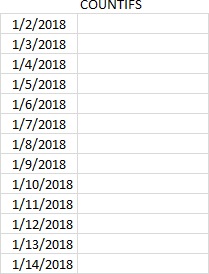The following formula returns an array of Excel dates needed to extract the missing dates.

ROW(INDIRECT(MIN(\$B\$3:\$C\$5)&":"&MAX(\$B\$3:\$C\$5)))

becomes

ROW(INDIRECT(43102&":"&43114))

becomes

ROW(43102:43114)

and returns

{43102; 43103; 43104; 43105; 43106; 43107; 43108; 43109; 43110; 43111; 43112; 43113; 43114}.

#### Step 6 - which dates are outside the date ranges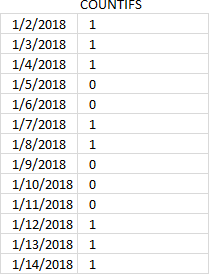The COUNTIFS function returns an array that we can use to extract dates not in date ranges. This particular COUNTIFS function has 4 arguments, however, you can use up to 255 arguments.

COUNTIFS(\$B\$3:\$B\$5, "<="&ROW(INDIRECT(MIN(\$B\$3:\$C\$5)&":"&MAX(\$B\$3:\$C\$5))), \$C\$3:\$C\$5, ">="&ROW(INDIRECT(MIN(\$B\$3:\$C\$5)&":"&MAX(\$B\$3:\$C\$5))))

becomes

COUNTIFS(\$B\$3:\$B\$5,{"<=43102"; "<=43103"; "<=43104"; "<=43105"; "<=43106"; "<=43107"; "<=43108"; "<=43109"; "<=43110"; "<=43111"; "<=43112"; "<=43113"; "<=43114"},\$C\$3:\$C\$5,{">=43102"; ">=43103"; ">=43104"; ">=43105"; ">=43106"; ">=43107"; ">=43108"; ">=43109"; ">=43110"; ">=43111"; ">=43112"; ">=43113"; ">=43114"})

and returns {1; 1; 1; 0; 0; 1; 1; 0; 0; 0; 1; 1; 1}. This array tells us which dates is in the array and which are not. 1 - yes, 0 (zero) - no. The position in this array is important to identify the corresponding date.

#### Step 7 - Compare each value in array with 0 (zero)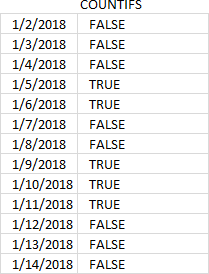Value 0 (zero) shows us that the corresponding date is not in the date range so I am now going to compare each value in the array to 0 (zero).

The equal sign lets you compare a value to an array of values, the result is a boolean value TRUE or FALSE.

COUNTIFS(\$B\$3:\$B\$5, "<="&ROW(INDIRECT(MIN(\$B\$3:\$C\$5)&":"&MAX(\$B\$3:\$C\$5))), \$C\$3:\$C\$5, ">="&ROW(INDIRECT(MIN(\$B\$3:\$C\$5)&":"&MAX(\$B\$3:\$C\$5))))=0

becomes

{1; 1; 1; 0; 0; 1; 1; 0; 0; 0; 1; 1; 1}=0

and returns

{FALSE; FALSE; FALSE; TRUE; TRUE; FALSE; FALSE; TRUE; TRUE; TRUE; FALSE; FALSE; FALSE}.

#### Step 8 - IF function returns an array of correct dates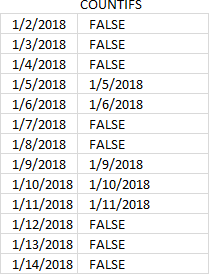The IF function uses the logical values to filter the dates we are looking for.

IF(COUNTIFS(\$B\$3:\$B\$5, "<="&ROW(INDIRECT(MIN(\$B\$3:\$C\$5)&":"&MAX(\$B\$3:\$C\$5))), \$C\$3:\$C\$5, ">="&ROW(INDIRECT(MIN(\$B\$3:\$C\$5)&":"&MAX(\$B\$3:\$C\$5))))=0, ROW(INDIRECT(MIN(\$B\$3:\$C\$5)&":"&MAX(\$B\$3:\$C\$5))))

becomes

IF({FALSE; FALSE; FALSE; TRUE; TRUE; FALSE; FALSE; TRUE; TRUE; TRUE; FALSE; FALSE; FALSE}, {43102; 43103; 43104; 43105; 43106; 43107; 43108; 43109; 43110; 43111; 43112; 43113; 43114})

and returns {FALSE; FALSE; FALSE; 43105; 43106; FALSE; FALSE; 43109; 43110; 43111; FALSE; FALSE; FALSE}

#### Step 9 - Extract the k-th smallest number (date)

The SMALL function returns dates based on their sizes, the second argument uses an expanding cell reference so that the small function extracts the smallest value in cell B8 and the second smallest in cell B9 and so on.

SMALL(IF(COUNTIFS(\$B\$3:\$B\$5, "<="&ROW(INDIRECT(MIN(\$B\$3:\$C\$5)&":"&MAX(\$B\$3:\$C\$5))), \$C\$3:\$C\$5, ">="&ROW(INDIRECT(MIN(\$B\$3:\$C\$5)&":"&MAX(\$B\$3:\$C\$5))))=0, ROW(INDIRECT(MIN(\$B\$3:\$C\$5)&":"&MAX(\$B\$3:\$C\$5)))), ROWS(\$A\$1:A1))

becomes

SMALL({FALSE; FALSE; FALSE; 43105; 43106; FALSE; FALSE; 43109; 43110; 43111; FALSE; FALSE; FALSE}, ROWS(\$A\$1:A1))

The ROWS function counts the number of rows in a given cell reference, the cell reference used here is a growing cell reference. It contains an absolute and a relative part indicated by the dollar signs.

becomes

SMALL({FALSE; FALSE; FALSE; 43105; 43106; FALSE; FALSE; 43109; 43110; 43111; FALSE; FALSE; FALSE}, 1)

and returns 43105 in cell B8.

Excel formats the number as a date and shows 1/5/2018, see picture below.Back to top

### Download Excel fileFind-missing-dates-in-a-set-of-date-rangesv2.xlsx

Back to top